## ﹏程序员的神话传说﹏﹏﹏﹏﹏﹏﹏﹏﹏﹏﹏﹏﹏﹏﹏

两亿年前的一天，很多巨大的陨石砸中地球大气层，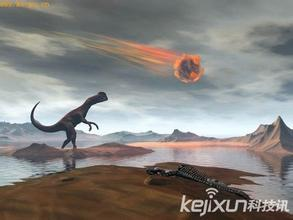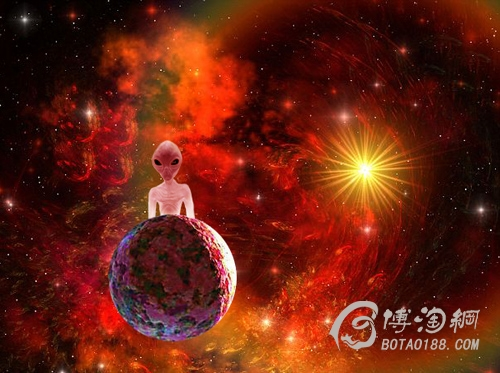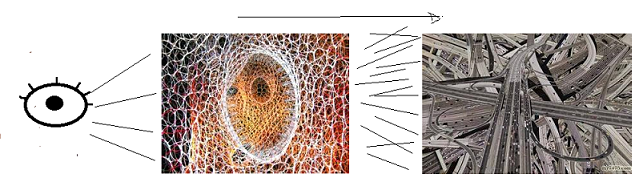))))))))))))))))))))))))))2亿年后，人类21世纪，中国---考古学家讨论研究室-------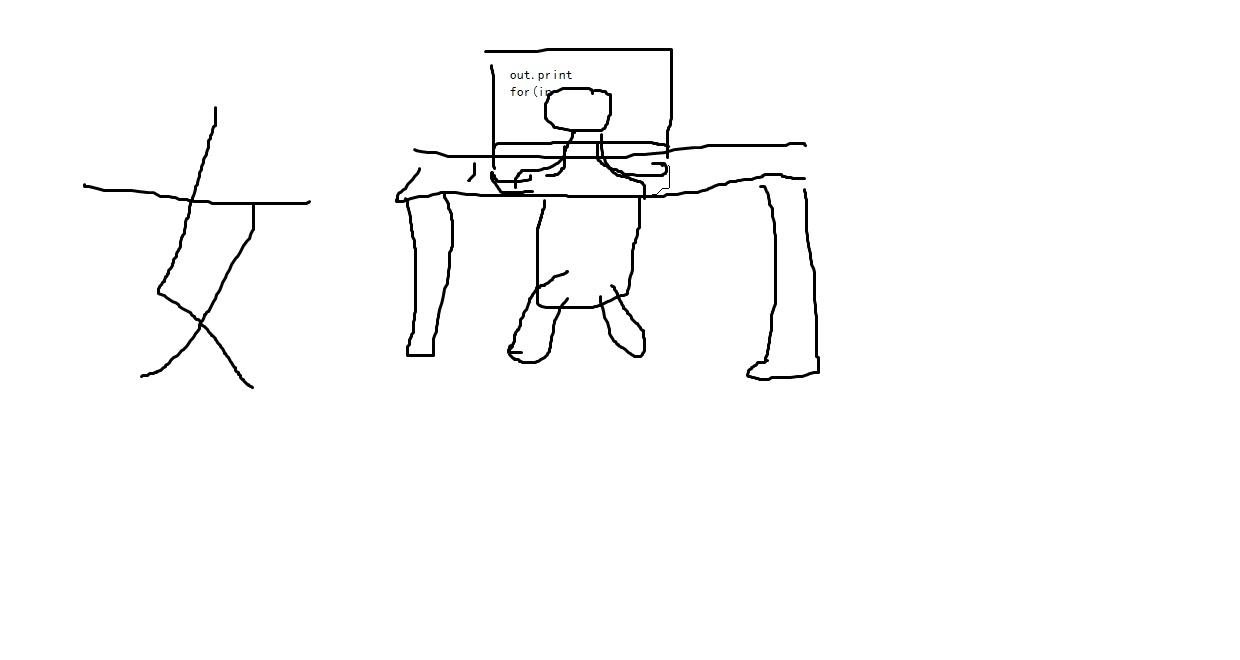0
M

M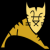M